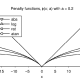A Coin Game

Recently, a friend of mine showed me a very interesting game with coins. She asked me to bring three saucers first and she placed them in a line. Then she placed 5 coins of different denominations, one on top of another in the first saucer.

The coins were of the denominations Re 1/-, 50P, 10P, 5P and 25P and she placed the coins in the order of their size—smallest on the top and biggest in the bottom.

She now asked me to transpose these coins to the third saucer observing the conditions that I transpose only one coin at a time, I do not place a bigger on a small one and I use the middle saucer only temporarily observing the first two conditions but that in the end the coins must be in third saucer and in the original order.

‘Oh that’s very simple. This hardly needs much effort’ I said.

I took the 25P coin and put it in the third saucer. Then I kept the 5P coin in the middle saucer. Now I got stuck. I did not know where to put the 10P coin. It was bigger then both!

My friend smiled and said ‘put the 25P coin on top of the 5P coin. Then you can put the 10P coin in the third saucer’.

I saw my way and did exactly what she told me. But to my great surprise I saw that my trouble had just begun. Where do I put 50P coin?

I did a lot of thinking. I put the 25P coin into the first saucer, the 5P coin into the third and transposed the 25P coin there two. And now I could place the 50P coin in the second saucer.

After numerous transpositions—at last—I was able to succeed in moving the entire pile of coins from the first saucer to the third.

How many movers did I make altogether?Vineet Patawari

Hi, I'm Vineet Patawari. I fell in love with numbers after being scared of them for quite some time. Now, I'm here to make you feel comfortable with numbers and help you get rid of Math Phobia!

4 thoughts to “A Coin Game”

1.Ameya says:

If u did it perfectly then 15 moves are enough watch rise of the planet of the apes the apes play same game

2.Chris says:

Notice that if you get the top 4 coins into the middle saucer, you can then move the biggest coin to the third saucer and then move the 4 coins on top of it.

If we let M(n) be the number of moves it takes to move n coins, then we get the following recurrence formula:
M(5) = M(4) + 1 + M(4) = 2M(4) + 1

This can actually be generalized to any number of coins. If you need to move a stack of n coins from saucer A to saucer B using saucer C as a temporary one in the middle, you can simply move n-1 coins to saucer C (which takes M(n-1) moves using saucer A as the source, saucer C as the destination, and saucer B as the temporary saucer in the middle) and then move the “n”th coin to the destination. Then move the n-1 coins back to saucer B (which takes M(n-1) moves using saucer C as the source, saucer B as the destination, and saucer A as the temporary saucer in the middle).
M(n) = 2M(n-1) +1

Since we know the solution for M(1) = 1, we can solve this equation.
In fact, M(n) = 2^n – 1, which can be proven rather easily by induction.
M(1) = 2^1 – 1 = 1 holds
Assume M(n) = 2^n -1
M(n+1) = 2M(n) + 1 = 2 (2^n – 1) + 1 = 2*2^n – 2 + 1 = 2^(n+1) – 1 holds
Thus, M(n) = 2^n -1 for all n>=1

Thus, with 5 coins, the number of moves will be M(5) = 2^5 – 1 = 31

1.Saptarshi pal chaudhuri says:

yup got d same answr d by induction…

3.mohit says:

30